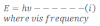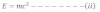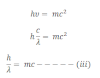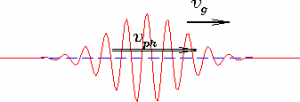# de-Broglie Hypothesis

According to de-Broglie hypothesis, the energy of the universe is in the form of matter and radiation. SO, both matter and radiation should have the similar properties. In fact radiation shows dual nature sometimes as wave and sometimes as particle. So, matter should have wave which can be expressed as:
Matter wave length = h/mv
where m is mass of particles/ matter
v= velocity and h is plank’s constant

The wavelength of the wave corresponding to matter is known as de-Broglie wavelength or matter wavelength.

Derivation of de-Broglie’s wavelength:
According to Plank’s quantum theory, energy of photon is expressed as:According to Einstein’s mass energy relation,Now from equation i and ii we get,This equation shows that wavelength depends upon mass and velocity of light i.e. Momentum of the particle.

If the particle of a mass m moves with velocity v then the matter wavelength can be expressed as which is known as de-Broglie wavelength.

Hence the de-Broglie wavelength rages between 0 and infinity.

The de-Broglie wavelength which is associated with the matter deals with uncertainty of the moving particles, problem arises to determine the exact location. In that sense , de –Broglie wavelength deals with uncertainty of particles. The diagram for de-Broglie wavelength is as shown in fig:Additionally, de-Broglie waves are not electromagnetic. It is because for the formation of electromagnetic waves, particle should be charged.